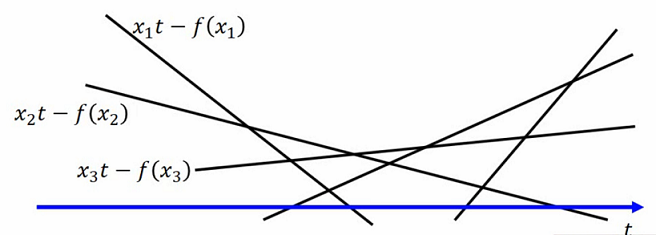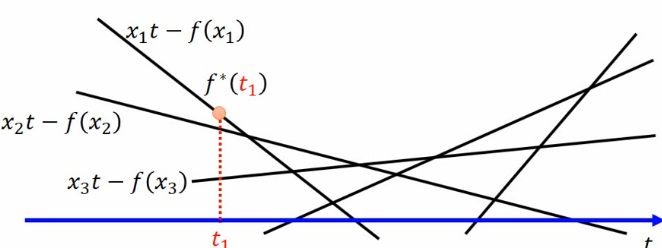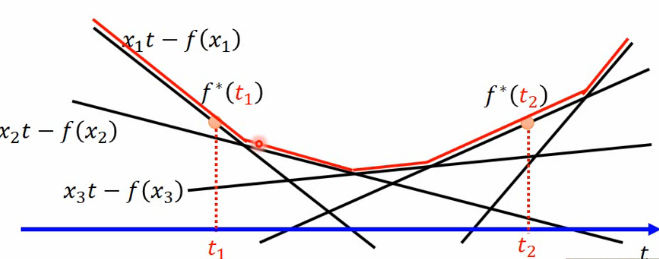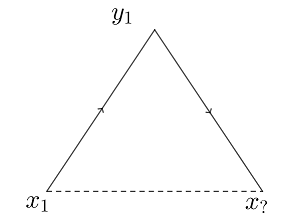# 共轭函数

$f ^ { * } ( t ) = \max _ { x \in \operatorname { dom } ( f ) } \{ x t - f ( x ) \}$

1.无论$f ( t )$是不是凸函数，$f ^ { * } ( t )$是凸函数。

2.凸函数的共轭函数的共轭函数是它自己。### 手机扫码访问

•